# KSEEB SSLC Class 10 Science Solutions Chapter 10 Light Reflection and Refraction

KSEEB SSLC Class 10 Science Solutions Chapter 10 Light Reflection and Refraction are part of KSEEB SSLC Class 10 Science Solutions. Here we have given Karnataka SSLC Class 10 Science Solutions Chapter 10 Light Reflection and Refraction.

## Karnataka SSLC Class 10 Science Solutions Chapter 10 Light Reflection and Refraction

### KSEEB SSLC Class 10 Science Chapter 10 Intext Questions

Text Book Part II Page No. 78

Question 1.
Define the principal focus of a concave mirror.
Answer:
The number of rays parallel to the principal axis are falling on a concave mirror which meat at a point is called principal focus of the concave mirror.

Question 2.
The radius of curvature of a spherical mirror is 20 cm. What is its focal length?
Answer:
R = 2f Here R = 20 cm
20 = 2f
∴ $$f=\frac { 20 }{ 2 } =10$$
∴ Focal length = 10 cm.

Question 3.
Name a mirror that can give an erect and enlarged image of an object.
Answer:
Concave mirror.

Question 4.
Why do we prefer a convex mirror as a rear-view mirror in vehicles?
Answer:
Because these mirrors are fitted on the sides of the vehicle, enabling the driver to see traffic behind him/her to facilitate safe driving.

Text Book Part II Page No. 81

Question 1.
Find the focal length of a convex mirror whose radius of curvature is 32 cm.
Answer:
Radius of curvature, R = 32 cm
Radius of curvature = 2f
$$R=2f=\frac { R }{ 2 } =\frac { 32 }{ 2 } =16$$
∴ Convex mirror focal length is = 16cm

Question 2.
A concave mirror produces three times magnified (enlarged) real image of an object placed at 10 cm in front of it. Where is the image located?
Answer:
$$M=\frac { Height\quad of\quad image }{ Height\quad of\quad object }$$
$$=\frac{h_{1}}{h_{0}}=\frac{-u}{v}$$
Let the height of object be h then height of image h = – 3h
$$=\frac{3 h}{h}=\frac{-v}{u}=\frac{v}{u}=3$$
∴ Distance of object, u = – 10 cm
v = 3 × (10) = – 30 cm
Here – sign indicates, image is real and it is 30 cm in front of concave mirror.

Text Book Part II Page No. 86

Question 1.
A ray of light traveling in air enters obliquely into water. Does the light ray bend towards the normal or away from the normal? Why?
Answer:
Lightray bend towards normal. Because when a ray of light enters from rearer medium to denser medium, it changes its direction in the second medium.

Question 2.
Light enters from air to glass having refractive index 1.50. What is the speed of light in the glass ? The speed of light in vaccum is 3 108 ms-1
Answer:
Refractive index, nm
$$=\frac { Velocity\quad of\quad light\quad in\quad vaccum\quad }{ Refractive\quad Index\quad of\quad glass }$$
$$=\frac{3 \times 10^{8}}{1.50}=2 \times 10^{8} \mathrm{m} / \mathrm{s}$$

Question 3.
Find out, from Tabel 10.3, the medium having highest optical density. Also find the medium with lowest optical density.
Answer:
Diamond is having highest optical density.
Air is having lowest optical density.

Question 4.
You are given kerosene, turpentine and water. In which of these does the light travel fastest ? Use the information given in Table 10.3
Answer:
Light travel faster in water because Refractive index of water is lesser than kerosene and turpentine.

Question 5.
The refractive index of diamond is 2.42. What is the meaning of this statement?
Answer:
It means Ratio of velocity of light in air and velocity of air in diamond is 2.42.

Text Book Part II Page No. 94

Question 1.
Define 1 dioptre of power of a lens.
Answer:
1 dioptre is the power of lens whose focal length is 1 metre 1 D = 1 m-1

Question 2.
A convex lens forms a real and inverted image of a needle at a distance of 50 cm from it. Where is the needle placed in front of the convex lens if the image is equal to the size of the object? Also, find the power of the lens.
Answer:
Image of Needle is real and inverted means this is real image it is 2f
Image is at a distance of 50 cm
Hence needle is kept 50 cm in front of convex lens.
Distance of object, u = – 50 cm.
Distance of image v = 50 cm
Focal length f = ?
As per lens formula.f = 25 cm = 0.25 m
Power of the lens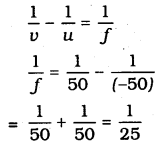Power of the lens P = + 4D.

### KSEEB SSLC Class 10 Science Chapter 10 Textbook Exercises

Question 1.
Which one of the following materials cannot be used to make a lens?
(a) Water
(b) Glass
(c) Plastic
(d) Clay
Answer:
(d) Clay.

Question 2.
The image formed by a concave mirror is observed to be virtual, erect and larger than the object. Where should be the position of the object?
(a) Between the principal focus and the centre of curvature
(b) At the centre of curvature
(c) Beyond the centre of curvature
(d) Between the pole of the mirror and its principal focus.
Answer:
(d) Between the pole of the mirror and its principal focus.

Question 3.
Where should an object be placed in front of a convex lens to get a real image of the size of the object?
(a) At the principal focus of the lens
(b) At twice the focal length
(c) At infinity
(d) Between the optical centre of the lens and its principal focus.
Answer:
(b) At twice the focal length.

Question 4.
A spherical mirror and a thin spherical lens have each a focal length of -15 cm. The mirror and the lens are likely to be
(a) both concave.
(b) both convex.
(c) the mirror is concave and the lens is convex.
(d) the mirror is convex, but the lens is concave.
Answer:
(a) both concave.

Question 6.
Which of the following lenses would you prefer to use while reading small letters found in a dictionary?
(a) A convex lens of focal length 50 cm.
(b) A concave lens of focal length 50 cm.
(c) A convex lens of focal length 5 cm.
(d) A concave lens of focal length 5 cm.
Answer:
(c) A convex lens of focal length 5cm.

Question 7.
We wish to obtain an erect image of an object, using a concave mirror of focal length 15 cm. What should be the range of distance of the object from the mirror?
What is the nature of the image? Is the image larger or smaller than the object?
Draw a ray diagram to show the image formation in this case.
Answer:
Distance of the object = o to 15 cm
Nature of image = virtual, erect and bigger than object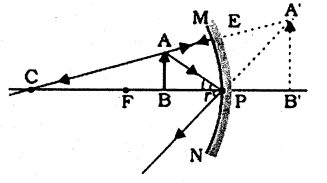Question 8.
Name the type of mirror used in the following situations.
(a) Headlights of a car.
(b) Side/rear-view mirror of a vehicle.
(c) Solar furnace.
Support your answer with reason.
Answer:
(a) Concave mirrors are used as reflectors in headlights of cars. When a bulb is located at the focus of the concave mirror, the light rays after reflection from the mirror travel over a large distance as a parallel beam of high intensity.
(b) A convex mirror is used as a side/ rear-view mirror of a vehicle because,

• A convex mirror always forms an erect, virtual, and diminished image of an object placed anywhere in front of it.
• A convex mirror has a wider field of view than a plane mirror of the same size.

(c) Large concave mirrors are used to concentrate sunlight to produce heat in solar furnaces.

Question 9.
One-half of a convex lens is covered with a black paper. Will this lens produce a complete image of the object? Verify your answer experimentally. Explain your observations.
Answer:
This lens gives full image, though one-half of this lens is covered with black paper as shown in below figure.As shown in figure light ray moves in half part and image is formed in another part of the lens.
If black paper is covered in lower part: Following figure explain thisQuestion 10.
An object 5 cm in length is held 25 cm away from a converging lens of focal length 10 cm. Draw the ray diagram and find the position, size and the nature of the image formed.
Answer:
Height of object, h = 5 cm
Distance of object from converging lens u = 25 cm
Focal length of lens f = 10 cm
As per lens formula $$\frac{1}{v}-\frac{1}{u}=\frac{1}{f}$$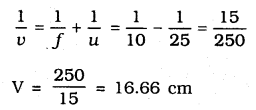Converging lens, $$\frac{h_{1}}{h_{0}}=\frac{v}{u}$$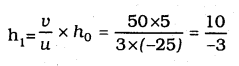= – 3.3 cm
Images is inverted and it is formed it is formed behind the lens about 16.7 cm. Its height is 3.3 cm.
Diagram is as follows: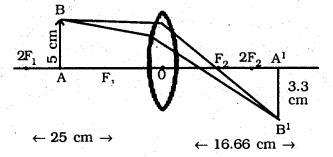Question 11.
A concave lens of focal length 15 cm forms an image 10 cm from the lens. How far is the object placed from the lens? Draw the ray diagram.
Answer:
Focal length (F1) of concave lens
f = 15 cm
Image distance, v = – 10 cm
As per lens formulau = -30 cm
Negative sign indicates, image is front of the lens about 30 cm.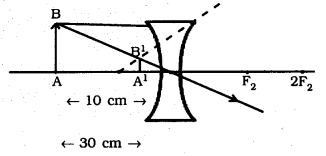Question 12.
An object is placed at a distance of 10 cm from a convex mirror of focal length 15 cm. Find the position and nature of the image.
Answer:
Focal length of convex mirror,
f = +15 cm
Object distance, u = -10 cm
As per lens formula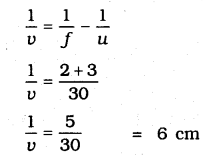Magnification $$=\frac{v}{u}=\frac{-6}{-10}=0.6$$
Virtual image is formed at the distance of 6 cm and it is erect.

Question 13.
The magnification produced by a plane mirror is +1. What does this mean?
Answer:
The positive [+] sign of magnification [m] indicates that the image is virtual and erect. The magnification m = 1 indicates that the image is of the same size as the object. Thus, the magnification of +1 produced by a plane mirror means the image formed in a plane mirror is virtual, erect and of the same size as the object.

Question 14.
An object 5.0 cm in length is placed at a distance of 20 cm in front of a convex mirror of radius of curvature 30 cm. Find the position of the image, its nature and size.
Answer:
Object distance, u = 20 cm
Height of object h = 5 cm
Radius of curvature R = 30 cm
R = 2f, f = 15 cm
As per mirror formulaPositive sign indicates image is formed behind the mirror
Magnification $$=\quad \frac { Image\quad distance }{ Object\quad distance }$$
$$=\frac{-8.57}{-20}=0.428$$
Image is behind the mirror because magnification is positive
Magnification $$=\frac{\text { Image distance }}{\text { Object distance }}$$
$$=\frac{h^{1}}{h}$$
h1 = m × h = 0.428 × 5 = 2.14 cm

Question 15.
An object of size 7.0 cm is placed at 27 cm in front of a concave mirror of focal length 18 cm. At what distance from the mirror should a screen be placed so that a sharply focussed image can be obtained? Find the size and nature of the image.
Answer:
Objective distance, u = 27 cm
Object height, h = 7 cm
Focal length, f = 30 cm
R = 2f, f = -18 cm
As per mirror formula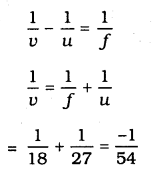Screen should be placed in front of mirror at the distance of = 54 cmNegative sign of magnification indicates image is real Magnification,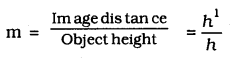h1 = 7 × (2) = -14 cm
Image is inverted because of negative sign.

Question 16.
Find the focal length of a lens of power – 2.0 D. What type of lens is this?
Answer:
Power of lens, P $$=\frac{1}{f}$$
P = -2D
f $$=\frac{-1}{2}=-0.5 \mathrm{cm}$$
Negative sign indicates this is concave lens.

Question 17.
A doctor has prescribed a corrective lens of power +1.5 D. Find the focal length of the lens. Is the prescribed lens diverging or converging?
Answer:
Power of lens, P $$=\frac{1}{f}$$
P = 1.5 D
F $$=\frac{1}{1.5}=\frac{10}{15}=0.66 \mathrm{m}$$
This is converging lens means convex lens.

### KSEEB SSLC Class 10 Science Chapter 10 Additional Questions and Answers

Question 1.
What are spherical mirrors?
Answer:
Mirrors, whose reflecting surfaces are spherical, are called spherical mirrors.

Question 2.
What is pole of the mirror?
Answer:
The centre of the reflecting surface of a spherical mirror is a point called the pole.

Question 3.
What is principal axis?
Answer:
The line passing through the pole and the centre of curvature of a spherical mirror is called principal axis.

Question 4.
Draw a ray diagram of concave mirror and convex mirror.
Answer: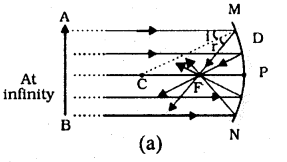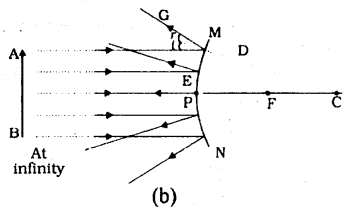(a) Concave mirror (b) Convex mirror

Question 5.
Draw a neat diagram showing Refraction of light through a rectangular glass slab
Answer :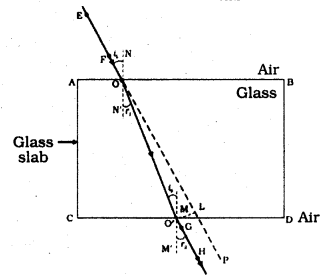We hope the given KSEEB SSLC Class 10 Science Solutions Chapter 9 Heredity and Evolution will help you. If you have any query regarding Karnataka SSLC Class 10 Science Solutions Chapter 9 Heredity and Evolution, drop a comment below and we will get back to you at the earliest.

error: Content is protected !!